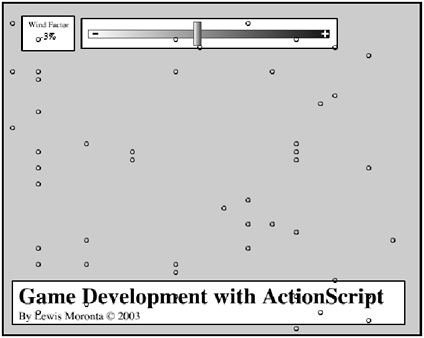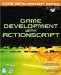# Working with Wind

 [ LiB ]

When I discussed particle systems I touched briefly on wind; this time, you will see wind in different light. I have prepared a demo for this chapter that allows you to play God and adjust the wind on-the-fly with a slider. You will see all of the falling particles move in the direction you push the slider, and in the amount you push it as well.

NOTE

NOTE

I'm going to start combining con cepts that I've taught you because at this point you should be comfortable enough with them.This is why the demos may seem more complicated. Inspect them and make sure you know how they work, and you will be able to make even cooler effects in your games .

Figure 12.7 shows the GDA_PROG12.4.fla demo, and how I set up the controls that enable you to control the program's wind factor.

##### Figure 12.7. Adjusting the wind factorI have embedded some of the code in the main Timeline and the rest of it within the slider Movie Clip. See the following listing for the code within the main Timeline.

` // Game Development with ActionScript // By Lewis Moronta (c) 2003 // This code segment shows you how // to setup particles that can // have an external force // affect them. onLoad = function () {   // Setup a new property   wind = 0;   // Create 50 new particles and place them randomly   for (var i = 0; i < 50; i++) {         // Create another one         attachMovie("circle", "circle"+i, i);         // Store the new Movie Clip temporarily         // to avoid any typos within the code         var mc = _root["circle"+i];         // Use the new alias to assign a pos          mc._x = Math.random()*550;         mc._y = Math.random()*400;   } }; onEnterFrame = function() {   // Loop through all of them   for (var i = 0; i < 50; i++) {          // Access the current Movie Clip          var mc = _root["circle"+i];          // Move the Movie Clip          mc._y += 10;          mc._x += wind;          //** Constrain the Movie Clip **//          // Loop it around the top          if (mc._y > 400)            mc._y = 0;          // Loop it around the right          if (mc._x > 500)            mc._x = 0;          // Loop it around the left          if (mc._x < 0)            mc._x = 500;    } }; `

Besides setting up an onLoad function, I also set up a wind variable. This will hold the direction that the wind should flow.

` onLoad = function () {   // Setup a new property   wind = 0; `

To get the particles on the stage, I need to link one of the symbols with the library and just duplicate the particles on the stage in different positions . I used the following loop for this effect:

` // Create 50 new particles and place them randomly for (var i = 0; i < 50; i++) {        // Create another one       attachMovie("circle", "circle"+i, i);       // Store the new Movie Clip temporarily       // to avoid any typos within the code       var mc = _root["circle"+i];       // Use the new alias to assign a pos       mc._x = Math.random()*550;       mc._y = Math.random()*400; } `

Besides creating animation on each frame, I also had to loop through all the pieces on the stage, so I added a for loop for my onEnterFrame handler:

` onEnterFrame = function() {   // Loop through all of them   for (var i = 0; i < 50; i++) { `

For sake of clarity, I stored the current Movie Clip being accessed into a small variablethis will allow me to skip writing out the whole reference during other parts of the code:

` // Access the current Movie Clip var mc = _root["circle"+i]; `

Once that was prepared, I had each particle falling at a constant 10 pixels per second, and I also added the wind factorthis value depends on where the slider is. It all will come together soon.

` // Move the Movie Clip mc._y += 10; mc._x += wind; `

I then made sure that if the particles go off the bottom of the stage that they loop around to the top of the stage.

` // Loop it around the top if (mc._y > 400)   mc._y = 0; `

I added the following two if statements because of the wind mechanism in the program. These statements will avoid any glitches and errors when the user goes crazy adjusting wind factors.

` // Loop it around the right if (mc._x > 500)   mc._x = 0;   // Loop it around the left if (mc._x < 0)     mc._x = 500; `

Now that you know how I created all the particles and how to have wind affect them, let's see how the user gets to control all this stuff. The following listing was extracted from the slider Movie Clip.

` // Game Development with ActionScript // By Lewis Moronta (c) 2003 // This clip contains slider code onClipEvent(load) {   // Setup min and max constraints   min = 100;   max = 400;   // Make sure it's not being clicked   active = false;   // Calculate how far along the slider   _root.display = "0%";   // Flag the program that the user released   onRelease = function () {     active = false;   };   // Flag the program the user released outside   onReleaseOutside = function () {     active = false;   };   // Flag the program the user clicked   onPress = function () {     active = true;   };   Mouse.addListener(this);   // Adjust the slider...   onMouseMove = function() {     // Only do this if this clip is pressed     if (active) {        // Follow the mouse on the x-axis       _x = _root._xmouse;       // Constrain motion        if (_x < min)         _x = min;       if (_x > max)         _x = max;       // More calculations =)       _root.wind = Math.round((_x-min)/(max-min)*60)-30;       _root.display = _root.wind+"%";     }   }; } `

What I wrote here can be intimidating at first because all the code is contained within the onClipEvent(load) handler.

In the beginning, I confidently set up two min and max variables that will act as my visual constrain positions. I also set up a flag variable that tells the program whether the user is dragging the slider or not. I also put a value into a dynamic text box that is on the _root .

` // Setup min and max constraints   min = 100;   max = 400;   // Make sure it's not being clicked   active = false;   // Calculate how far along the slider   _root.display = "0%"; `

Then, I flagged to see whether the user released the slider:

` // Flag the program that the user released onRelease = function () {   active = false; }; `

There was also the possibility of the user releasing outside the slider button, so I covered that, too.

` onReleaseOutside = function () {   active = false; }; `

I flagged the rest of the program for when the user presses and drags the slider.

` onPress = function () {   active = true; }; `

The next thing I did was add a mouse listener to this Movie Clip so that I can use the onMouseMove callback function:

` Mouse.addListener(this); `

Once in the onMouseMove function, I decided not to do anything if the slider wasn't active or being dragged, so I tested for that state:

` onMouseMove = function() { `

` // Only do this if this clip is pressed if (active) { `

You of course have to follow the cursor position; that's why I added the following line:

` // Follow the mouse on the x-axis       _x = _root._xmouse; `

In order to allow the user to only move the slider within certain parts of the stage, you must set up constraints, so that's exactly what I did:

` // Constrain motion       if (_x < min)        _x = min;       if (_x > max)         _x = max; `

The following line is the juiciest part of this code. It calculates the position of the slider and, from this, evaluates the strength and direction of the wind (there's a vector value for ya).

` _root.wind = Math.round((_x-min)/(max-min)*60)-30; `

This works because I divided the portion (the number of pixels along the slider) by the whole (the total length of the slider) to get a percentage amount in decimals. I multiplied by 60 to scale the value to a range of 0 to 60. I then subtracted 30 to get a new range of 30 to 30. In other words, when the slider is all the way to the left, it will yield 30. When the slider is all the way to the right, the max it can go is 30. When the slider is in the center, it will be 0.

And finally, for display purposes, I update the dynamic textbox that exists in the main Timeline.

` _root.display = _root.wind+"%"; `

How do you like the interactivity now?

 [ LiB ]Game Development with ActionScript
ISBN: 1592001106
EAN: 2147483647
Year: 2004
Pages: 162
Authors: Lewis Moronta